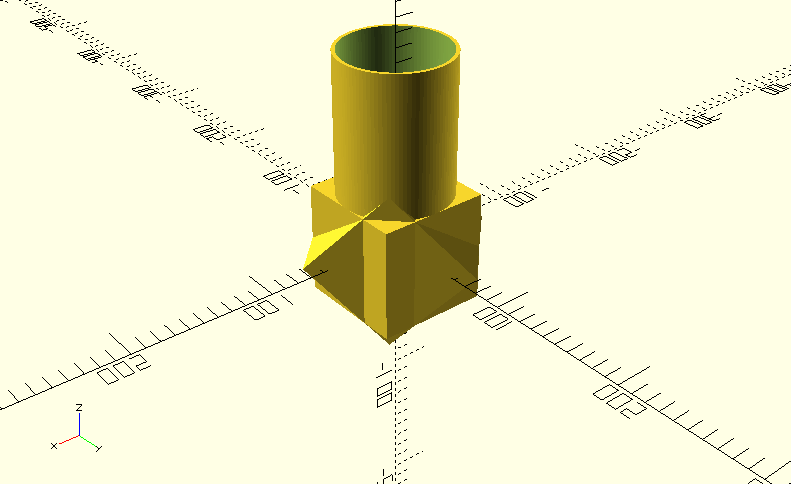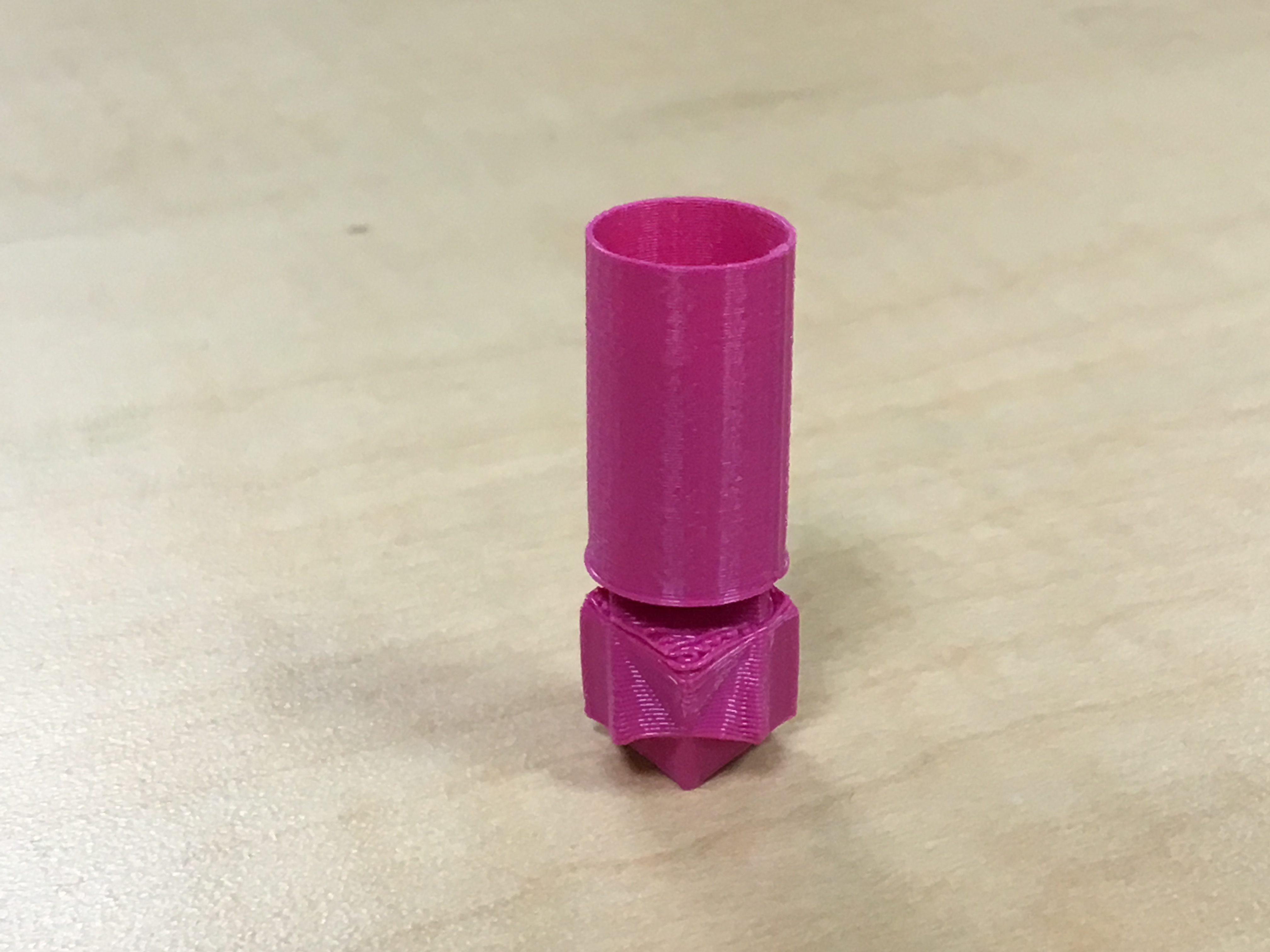After starting this project, I quickly realized that it was not going to be easy. I struggled for many hours trying to figure out how to write code using the material and instructions provided for us and could not figure it out. I decided that watching someone else write code may be helpful to me, so I decided to watch some YouTube tutorials. After watching few, I decided to combined two designs I learned to make from watching different YouTube tutorials. for my first simple Openscad design, I made a simple cup. To make this cup, you simple write code for a cylinder and then create another cylinder to make a whole for the cup.

### First Code: Cup

```\$fn=100;

cylinder(h=30,d=20, center);
difference() {
cylinder(d=80, h=140);
translate([0,0,5]) cylinder(d=75,h=150);}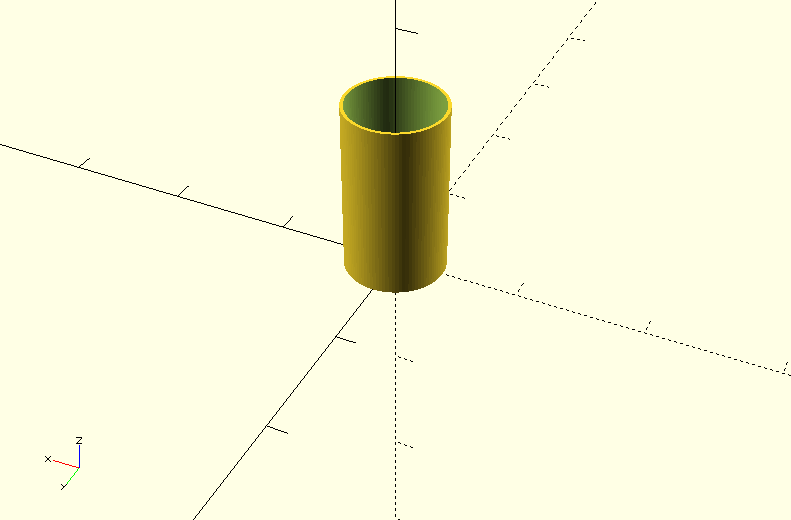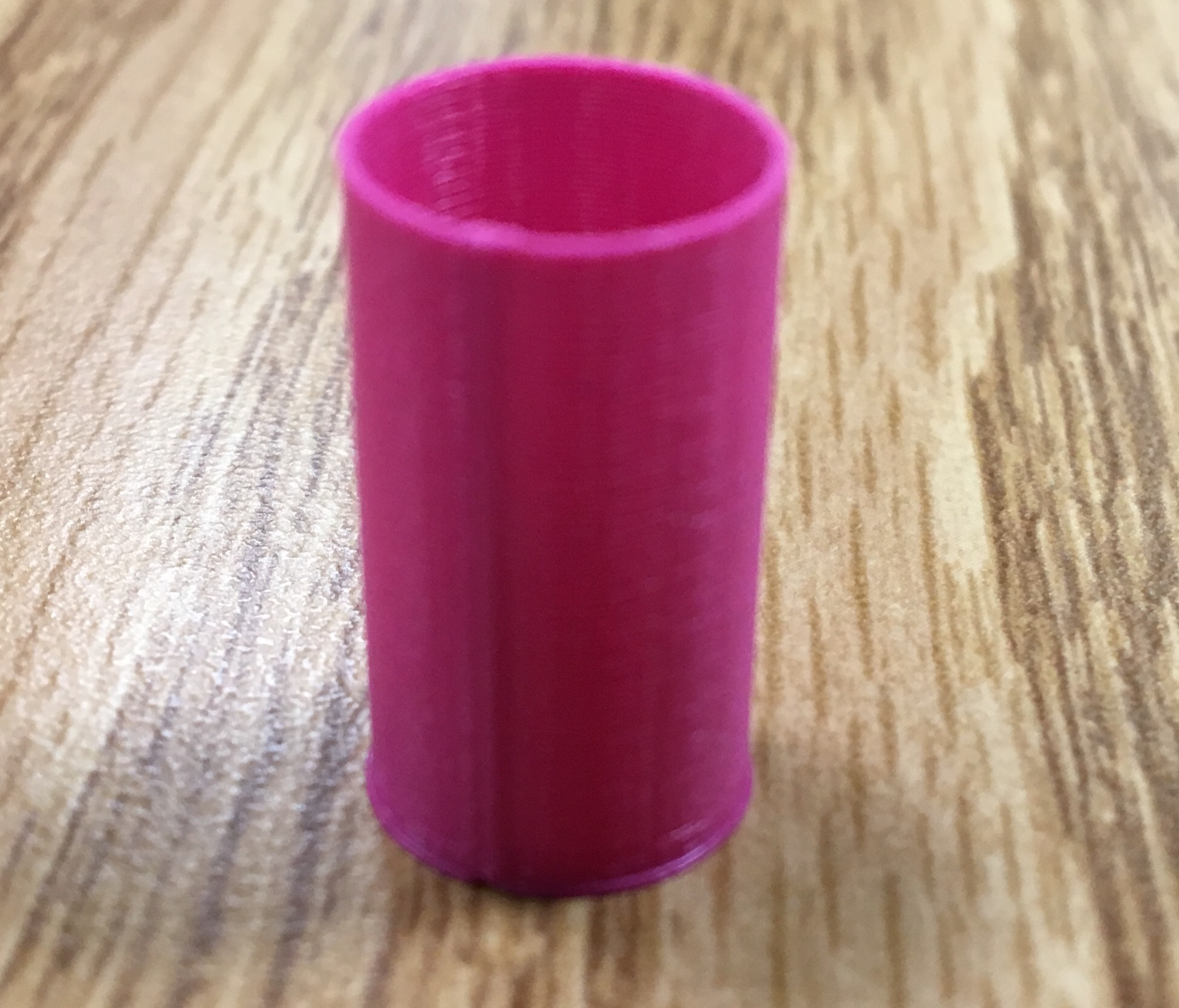```

I then created an eight-sided star by rotating and combining two cubes. To make my cup more interesting, I then combined the two codes into one and made the eight-sided star the platform for the cup.

### \$fn=100;

cylinder(h=30,d=20, center);
difference() {
cylinder(d=80, h=140);
translate([0,0,5]) cylinder(d=75,h=150);//}

//Eight-sided star
union(){
cube([80,80,80], center=true);
rotate([45,0,45]) cube([80,80,80], center=true);}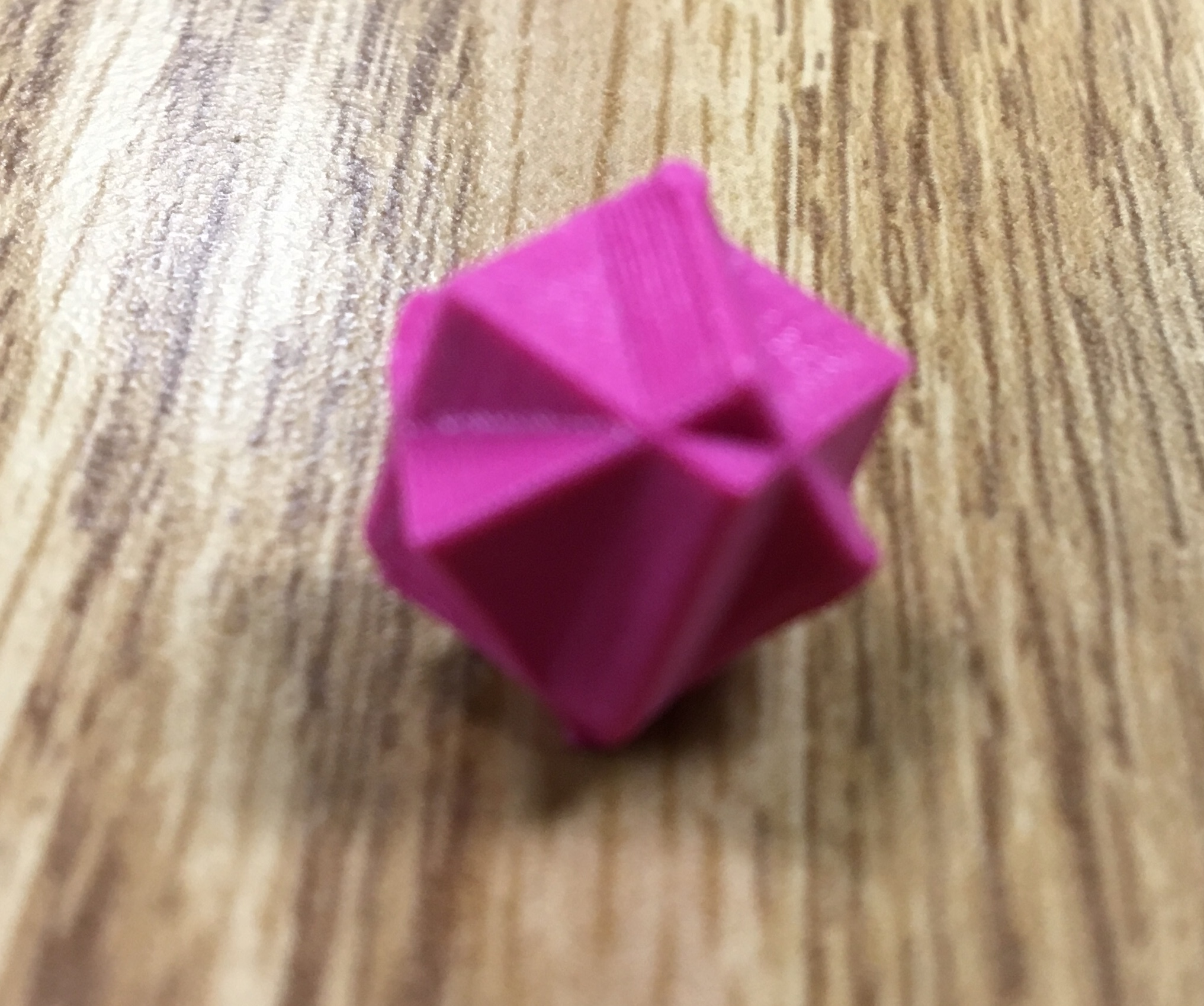## Final Product: